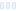# Formula L Biscuit Mix

#### Southern Biscuit - Walmart

Main info:

Formula L Biscuit Mix
Southern Biscuit - Walmart
1 serving
130.0 calories
17.0 grams carbs
6 grams fat
2.0 grams protein

1.0 grams fiber
0 mg cholesterol
1.5 grams saturated fat
420 mg sodium
0 grams sugar
0 grams trans fat

Nutrition Facts
For a Serving Size of (g)
How many calories are in Formula L Biscuit Mix? Amount of calories in Formula L Biscuit Mix: Calories Calories from Fat (%)
% Daily Value *
How much fat is in Formula L Biscuit Mix? Amount of fat in Formula L Biscuit Mix: Total Fat
How much saturated fat is in Formula L Biscuit Mix? Amount of saturated fat in Formula L Biscuit Mix: Saturated fat
How much sodium is in Formula L Biscuit Mix? Amount of sodium in Formula L Biscuit Mix: Sodium
How many carbs are in Formula L Biscuit Mix? Amount of carbs in Formula L Biscuit Mix: Carbohydrates
How many net carbs are in Formula L Biscuit Mix? Amount of net carbs in Formula L Biscuit Mix: Net carbs
How much fiber is in Formula L Biscuit Mix? Amount of fiber in Formula L Biscuit Mix: Fiber
How much glucose is in Formula L Biscuit Mix? Amount of glucose in Formula L Biscuit Mix: Glucose
How much protein is in Formula L Biscuit Mix? Amount of protein in Formula L Biscuit Mix: Protein
Vitamins and minerals
How much Vitamin A is in Formula L Biscuit Mix? Amount of Vitamin A in Formula L Biscuit Mix: Vitamin A
How much Vitamin C is in Formula L Biscuit Mix? Amount of Vitamin C in Formula L Biscuit Mix: Vitamin C
How much Calcium is in Formula L Biscuit Mix? Amount of Calcium in Formula L Biscuit Mix: Calcium
How much Iron is in Formula L Biscuit Mix? Amount of Iron in Formula L Biscuit Mix: Iron
Fatty acids
Amino acids
* The Percent Daily Values are based on a 2,000 calorie diet, so your values may change depending on your calorie needs.
Percent calories from...
##### Try our free Diet GeneratorI want to eat calories
in meals.
I like to eatLoading similar foods...
Note: Any items purchased after clicking our Amazon buttons will give us a little referral bonus. If you do click them, thank you!#### Try our Automatic Meal PlannerBe cool

- Zen orangeI never skip arm day

- Buff broccoli